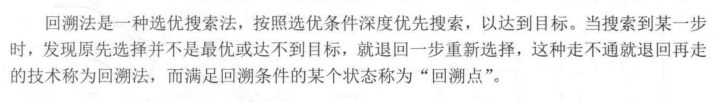# js回溯法计算最佳旅行线路代码实例_飛雲_前端开发者```var g = [
[Infinity,3    ,Infinity,8    ,9],
[ 3   ,Infinity,3    ,10   ,5],
[Infinity, 3   ,Infinity,4    ,3],
[8    ,10   ,4    ,Infinity,20],
[9    ,5    ,3    ,20   ,Infinity]
]```

```var g = [
[Infinity,3    ,Infinity,8    ,9],
[ 3   ,Infinity,3    ,10   ,5],
[Infinity, 3   ,Infinity,4    ,3],
[8    ,10   ,4    ,Infinity,20],
[9    ,5    ,3    ,20   ,Infinity]
]

var x = [0,1,2,3,4]; //城市的编号
var cl = 0;     //规划过程中记录的距离
var bestl = Infinity; //当前最优解
var bestx = [0,0,0,0,0]; //当前最优解的路径
//var t = 0; //当前需要到达的城市
var n = x.length-1;
function Traveling(t){
if(t > n ){
//搜索到底部，如果满足最优解则记录
if(g[x[n]] < Infinity && (cl + g[x[n]] < bestl)){
for(var j = 0; j <= n; j++){
bestx[j] = x[j];
}
bestl = cl + g[x[n]];
}
}else{
for(var j = t ; j <= n; j++){
if(g[x[t-1]][x[j]] < Infinity && (cl + g[x[t-1]][x[j]] < bestl )){
swap(x,t,j);        //交换位置，将j点作为 当前需要到达的城市
cl = cl + g[x[t-1]][x[t]]; //加上选中的点
Traveling(t+1);       //搜索下一下节点
cl = cl - g[x[t-1]][x[t]]; //还原搜索之前
swap(x,t,j);        //还原
}
}
}
}
function swap(arr,x,y){
var temp = arr[x];
arr[x] = arr[y];
arr[y] = temp;
}
Traveling(1);
console.log(bestx);
console.log(bestl)```

64K